# semanticseg

Semantic image segmentation using deep learning

## Syntax

``C = semanticseg(I,network)``
``[C,score,allScores] = semanticseg(I,network)``
``[___] = semanticseg(I,network,roi)``
``pxds = semanticseg(ds,network)``
``[___] = semanticseg(___,Name,Value)``

## Description

example

````C = semanticseg(I,network)` returns a semantic segmentation of the input image using deep learning.```
````[C,score,allScores] = semanticseg(I,network)` also returns the classification scores for each categorical label in `C`. The function returns the scores in an array that corresponds to each pixel or voxel in the input image.```
````[___] = semanticseg(I,network,roi)` returns a semantic segmentation for a rectangular subregion of the input image.```
````pxds = semanticseg(ds,network)` returns the semantic segmentation for a collection of images in `ds`, a datastore object.The function supports parallel computing using multiple MATLAB® workers. You can enable parallel computing using the Computer Vision Toolbox Preferences dialog.```
````[___] = semanticseg(___,Name,Value)` returns semantic segmentation with additional options specified by one or more name-value pair arguments.```

## Examples

collapse all

Overlay segmentation results on an image and display the results.

```data = load('triangleSegmentationNetwork'); net = data.net```
```net = SeriesNetwork with properties: Layers: [10x1 nnet.cnn.layer.Layer] InputNames: {'imageinput'} OutputNames: {'classoutput'} ```

List the network layers.

`net.Layers`
```ans = 10x1 Layer array with layers: 1 'imageinput' Image Input 32x32x1 images with 'zerocenter' normalization 2 'conv_1' Convolution 64 3x3x1 convolutions with stride [1 1] and padding [1 1 1 1] 3 'relu_1' ReLU ReLU 4 'maxpool' Max Pooling 2x2 max pooling with stride [2 2] and padding [0 0 0 0] 5 'conv_2' Convolution 64 3x3x64 convolutions with stride [1 1] and padding [1 1 1 1] 6 'relu_2' ReLU ReLU 7 'transposed-conv' Transposed Convolution 64 4x4x64 transposed convolutions with stride [2 2] and cropping [1 1 1 1] 8 'conv_3' Convolution 2 1x1x64 convolutions with stride [1 1] and padding [0 0 0 0] 9 'softmax' Softmax softmax 10 'classoutput' Pixel Classification Layer Class weighted cross-entropy loss with classes 'triangle' and 'background' ```

Read and display the test image.

```I = imread('triangleTest.jpg'); figure imshow(I)```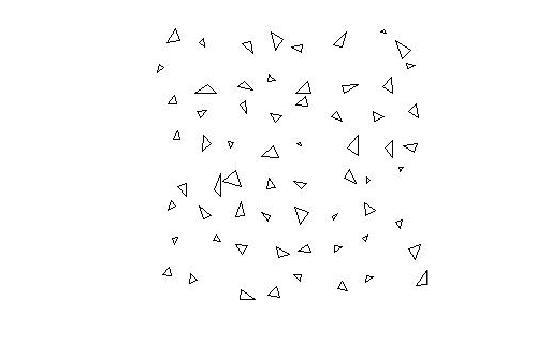Perform semantic image segmentation.

`[C,scores] = semanticseg(I,net,'MiniBatchSize',32);`

Overlay segmentation results on the image and display the results.

```B = labeloverlay(I, C); figure imshow(B)```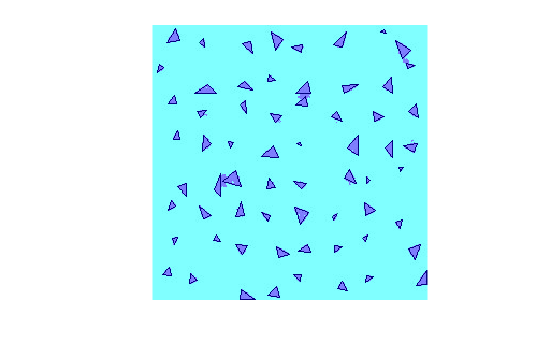Display the classification scores.

```figure imagesc(scores) axis square colorbar```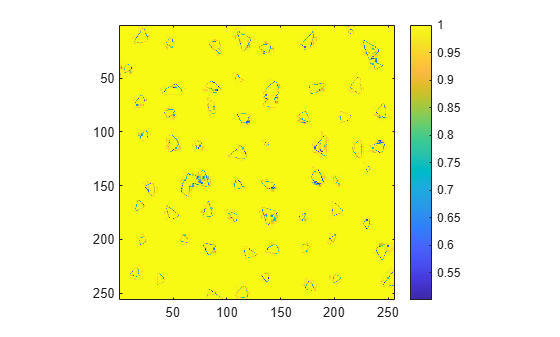Create a binary mask with only the triangles.

```BW = C == 'triangle'; figure imshow(BW)```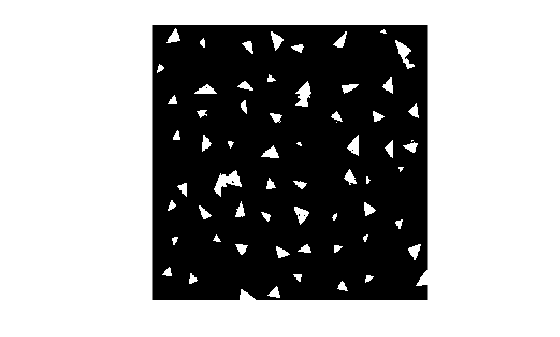Run semantic segmentation on a test set of images and compare the results against ground truth data.

```data = load('triangleSegmentationNetwork'); net = data.net;```

Load test images using `imageDatastore`.

```dataDir = fullfile(toolboxdir('vision'),'visiondata','triangleImages'); testImageDir = fullfile(dataDir,'testImages'); imds = imageDatastore(testImageDir)```
```imds = ImageDatastore with properties: Files: { ' .../toolbox/vision/visiondata/triangleImages/testImages/image_001.jpg'; ' .../toolbox/vision/visiondata/triangleImages/testImages/image_002.jpg'; ' .../toolbox/vision/visiondata/triangleImages/testImages/image_003.jpg' ... and 97 more } Folders: { ' .../build/matlab/toolbox/vision/visiondata/triangleImages/testImages' } AlternateFileSystemRoots: {} ReadSize: 1 Labels: {} SupportedOutputFormats: ["png" "jpg" "jpeg" "tif" "tiff"] DefaultOutputFormat: "png" ReadFcn: @readDatastoreImage ```

```testLabelDir = fullfile(dataDir,'testLabels'); classNames = ["triangle" "background"]; pixelLabelID = [255 0]; pxdsTruth = pixelLabelDatastore(testLabelDir,classNames,pixelLabelID);```

Run semantic segmentation on all of the test images with a batch size of 4. You can increase the batch size to increase throughput based on your systems memory resources.

`pxdsResults = semanticseg(imds,net,'MiniBatchSize',4,'WriteLocation',tempdir);`
```Running semantic segmentation network ------------------------------------- * Processed 100 images. ```

Compare the results against the ground truth.

`metrics = evaluateSemanticSegmentation(pxdsResults,pxdsTruth)`
```Evaluating semantic segmentation results ---------------------------------------- * Selected metrics: global accuracy, class accuracy, IoU, weighted IoU, BF score. * Processed 100 images. * Finalizing... Done. * Data set metrics: GlobalAccuracy MeanAccuracy MeanIoU WeightedIoU MeanBFScore ______________ ____________ _______ ___________ ___________ 0.90624 0.95085 0.61588 0.87529 0.40652 ```
```metrics = semanticSegmentationMetrics with properties: ConfusionMatrix: [2x2 table] NormalizedConfusionMatrix: [2x2 table] DataSetMetrics: [1x5 table] ClassMetrics: [2x3 table] ImageMetrics: [100x5 table] ```

This example shows how to define and create a custom pixel classification layer that uses Tversky loss.

This layer can be used to train semantic segmentation networks. To learn more about creating custom deep learning layers, see Define Custom Deep Learning Layers (Deep Learning Toolbox).

Tversky Loss

The Tversky loss is based on the Tversky index for measuring overlap between two segmented images . The Tversky index ${\mathrm{TI}}_{\mathit{c}}$ between one image $\mathit{Y}$ and the corresponding ground truth $\mathit{T}$ is given by

`${\mathrm{TI}}_{\mathit{c}}=\frac{{\sum }_{\mathit{m}=1}^{\mathit{M}}{\mathit{Y}}_{\mathrm{cm}}{\mathit{T}}_{\mathrm{cm}}}{{\sum }_{\mathit{m}=1}^{\mathit{M}}{\mathit{Y}}_{\mathrm{cm}}{\mathit{T}}_{\mathrm{cm}}+\alpha {\sum }_{\mathit{m}=1}^{\mathit{M}}{\mathit{Y}}_{\mathrm{cm}}{\mathit{T}}_{\stackrel{‾}{\mathrm{c}}\mathit{m}}+\beta {\sum }_{\mathit{m}=1}^{\mathit{M}}{\mathit{Y}}_{\stackrel{‾}{\mathrm{c}}\mathit{m}}{\mathit{T}}_{\mathrm{cm}}}$`

• $\mathit{c}$ corresponds to the class and $\stackrel{‾}{\mathit{c}}\text{\hspace{0.17em}}$corresponds to not being in class $\mathit{c}$.

• $\mathit{M}$ is the number of elements along the first two dimensions of $\mathit{Y}$.

• $\alpha$ and $\beta$ are weighting factors that control the contribution that false positives and false negatives for each class make to the loss.

The loss $\mathit{L}\text{\hspace{0.17em}}$over the number of classes $\mathit{C}$ is given by

`$\mathit{L}=\sum _{\mathit{c}=1}^{\mathit{C}}1-{\mathrm{TI}}_{\mathit{c}}$`

Classification Layer Template

Copy the classification layer template into a new file in MATLAB®. This template outlines the structure of a classification layer and includes the functions that define the layer behavior. The rest of the example shows how to complete the `tverskyPixelClassificationLayer`.

```classdef tverskyPixelClassificationLayer < nnet.layer.ClassificationLayer properties % Optional properties end methods function loss = forwardLoss(layer, Y, T) % Layer forward loss function goes here end end end ```

Declare Layer Properties

By default, custom output layers have the following properties:

• `Name` – Layer name, specified as a character vector or a string scalar. To include this layer in a layer graph, you must specify a nonempty unique layer name. If you train a series network with this layer and `Name` is set to `''`, then the software automatically assigns a name at training time.

• `Description` – One-line description of the layer, specified as a character vector or a string scalar. This description appears when the layer is displayed in a `Layer` array. If you do not specify a layer description, then the software displays the layer class name.

• `Type` – Type of the layer, specified as a character vector or a string scalar. The value of `Type` appears when the layer is displayed in a `Layer` array. If you do not specify a layer type, then the software displays `'Classification layer'` or `'Regression layer'`.

Custom classification layers also have the following property:

• `Classes` – Classes of the output layer, specified as a categorical vector, string array, cell array of character vectors, or `'auto'`. If `Classes` is `'auto'`, then the software automatically sets the classes at training time. If you specify a string array or cell array of character vectors `str`, then the software sets the classes of the output layer to `categorical(str,str)`. The default value is `'auto'`.

If the layer has no other properties, then you can omit the `properties` section.

The Tversky loss requires a small constant value to prevent division by zero. Specify the property, `Epsilon`, to hold this value. It also requires two variable properties A`lpha` and `Beta` that control the weighting of false positives and false negatives, respectively.

```classdef tverskyPixelClassificationLayer < nnet.layer.ClassificationLayer properties(Constant) % Small constant to prevent division by zero. Epsilon = 1e-8; end properties % Default weighting coefficients for false positives and false negatives Alpha = 0.5; Beta = 0.5; end ... end ```

Create Constructor Function

Create the function that constructs the layer and initializes the layer properties. Specify any variables required to create the layer as inputs to the constructor function.

Specify an optional input argument name to assign to the `Name` property at creation.

```function layer = tverskyPixelClassificationLayer(name, alpha, beta) % layer = tverskyPixelClassificationLayer(name) creates a Tversky % pixel classification layer with the specified name. % Set layer name layer.Name = name; % Set layer properties layer.Alpha = alpha; layer.Beta = beta; % Set layer description layer.Description = 'Tversky loss'; end ```

Create Forward Loss Function

Create a function named `forwardLoss` that returns the weighted cross entropy loss between the predictions made by the network and the training targets. The syntax for `forwardLoss` is `loss = forwardLoss(layer,Y,T)`, where `Y` is the output of the previous layer and `T` represents the training targets.

For semantic segmentation problems, the dimensions of `T` match the dimension of `Y`, where `Y` is a 4-D array of size `H`-by-`W`-by-`K`-by-`N`, where `K` is the number of classes, and `N` is the mini-batch size.

The size of `Y` depends on the output of the previous layer. To ensure that `Y` is the same size as `T`, you must include a layer that outputs the correct size before the output layer. For example, to ensure that `Y` is a 4-D array of prediction scores for `K` classes, you can include a fully connected layer of size `K` or a convolutional layer with `K` filters followed by a softmax layer before the output layer.

```function loss = forwardLoss(layer, Y, T) % loss = forwardLoss(layer, Y, T) returns the Tversky loss between % the predictions Y and the training targets T. Pcnot = 1-Y; Gcnot = 1-T; TP = sum(sum(Y.*T,1),2); FP = sum(sum(Y.*Gcnot,1),2); FN = sum(sum(Pcnot.*T,1),2); numer = TP + layer.Epsilon; denom = TP + layer.Alpha*FP + layer.Beta*FN + layer.Epsilon; % Compute Tversky index lossTIc = 1 - numer./denom; lossTI = sum(lossTIc,3); % Return average Tversky index loss N = size(Y,4); loss = sum(lossTI)/N; end ```

Backward Loss Function

As the `forwardLoss` function fully supports automatic differentiation, there is no need to create a function for the backward loss.

For a list of functions that support automatic differentiation, see List of Functions with dlarray Support (Deep Learning Toolbox).

Completed Layer

The completed layer is provided in `tverskyPixelClassificationLayer.m`.

```classdef tverskyPixelClassificationLayer < nnet.layer.ClassificationLayer % This layer implements the Tversky loss function for training % semantic segmentation networks. % References % Salehi, Seyed Sadegh Mohseni, Deniz Erdogmus, and Ali Gholipour. % "Tversky loss function for image segmentation using 3D fully % convolutional deep networks." International Workshop on Machine % Learning in Medical Imaging. Springer, Cham, 2017. % ---------- properties(Constant) % Small constant to prevent division by zero. Epsilon = 1e-8; end properties % Default weighting coefficients for False Positives and False % Negatives Alpha = 0.5; Beta = 0.5; end methods function layer = tverskyPixelClassificationLayer(name, alpha, beta) % layer = tverskyPixelClassificationLayer(name, alpha, beta) creates a Tversky % pixel classification layer with the specified name and properties alpha and beta. % Set layer name. layer.Name = name; layer.Alpha = alpha; layer.Beta = beta; % Set layer description. layer.Description = 'Tversky loss'; end function loss = forwardLoss(layer, Y, T) % loss = forwardLoss(layer, Y, T) returns the Tversky loss between % the predictions Y and the training targets T. Pcnot = 1-Y; Gcnot = 1-T; TP = sum(sum(Y.*T,1),2); FP = sum(sum(Y.*Gcnot,1),2); FN = sum(sum(Pcnot.*T,1),2); numer = TP + layer.Epsilon; denom = TP + layer.Alpha*FP + layer.Beta*FN + layer.Epsilon; % Compute tversky index lossTIc = 1 - numer./denom; lossTI = sum(lossTIc,3); % Return average tversky index loss. N = size(Y,4); loss = sum(lossTI)/N; end end end ```

GPU Compatibility

The MATLAB functions used in `forwardLoss` in `tverskyPixelClassificationLayer` all support `gpuArray` inputs, so the layer is GPU compatible.

Check Output Layer Validity

Create an instance of the layer.

`layer = tverskyPixelClassificationLayer('tversky',0.7,0.3);`

Check the validity of the layer by using `checkLayer` (Deep Learning Toolbox). Specify the valid input size to be the size of a single observation of typical input to the layer. The layer expects a `H`-by-`W`-by-`K`-by-`N` array inputs, where `K` is the number of classes, and `N` is the number of observations in the mini-batch.

```numClasses = 2; validInputSize = [4 4 numClasses]; checkLayer(layer,validInputSize, 'ObservationDimension',4)```
```Skipping GPU tests. No compatible GPU device found. Skipping code generation compatibility tests. To check validity of the layer for code generation, specify the 'CheckCodegenCompatibility' and 'ObservationDimension' options. Running nnet.checklayer.TestOutputLayerWithoutBackward ........ Done nnet.checklayer.TestOutputLayerWithoutBackward __________ Test Summary: 8 Passed, 0 Failed, 0 Incomplete, 2 Skipped. Time elapsed: 1.2631 seconds. ```

The test summary reports the number of passed, failed, incomplete, and skipped tests.

Use Custom Layer in Semantic Segmentation Network

Create a semantic segmentation network that uses the `tverskyPixelClassificationLayer`.

```layers = [ imageInputLayer([32 32 1]) convolution2dLayer(3,64,'Padding',1) batchNormalizationLayer reluLayer maxPooling2dLayer(2,'Stride',2) convolution2dLayer(3,64,'Padding',1) reluLayer transposedConv2dLayer(4,64,'Stride',2,'Cropping',1) convolution2dLayer(1,2) softmaxLayer tverskyPixelClassificationLayer('tversky',0.3,0.7)]```
```layers = 11x1 Layer array with layers: 1 '' Image Input 32x32x1 images with 'zerocenter' normalization 2 '' Convolution 64 3x3 convolutions with stride [1 1] and padding [1 1 1 1] 3 '' Batch Normalization Batch normalization 4 '' ReLU ReLU 5 '' Max Pooling 2x2 max pooling with stride [2 2] and padding [0 0 0 0] 6 '' Convolution 64 3x3 convolutions with stride [1 1] and padding [1 1 1 1] 7 '' ReLU ReLU 8 '' Transposed Convolution 64 4x4 transposed convolutions with stride [2 2] and cropping [1 1 1 1] 9 '' Convolution 2 1x1 convolutions with stride [1 1] and padding [0 0 0 0] 10 '' Softmax softmax 11 'tversky' Classification Output Tversky loss ```

Load training data for semantic segmentation using `imageDatastore` and `pixelLabelDatastore`.

```dataSetDir = fullfile(toolboxdir('vision'),'visiondata','triangleImages'); imageDir = fullfile(dataSetDir,'trainingImages'); labelDir = fullfile(dataSetDir,'trainingLabels'); imds = imageDatastore(imageDir); classNames = ["triangle" "background"]; labelIDs = [255 0]; pxds = pixelLabelDatastore(labelDir, classNames, labelIDs);```

Associate the image and pixel label data by using `pixelLabelImageDatastore`.

`ds = pixelLabelImageDatastore(imds,pxds);`

Set the training options and train the network.

```options = trainingOptions('adam', ... 'InitialLearnRate',1e-3, ... 'MaxEpochs',100, ... 'LearnRateDropFactor',5e-1, ... 'LearnRateDropPeriod',20, ... 'LearnRateSchedule','piecewise', ... 'MiniBatchSize',50); net = trainNetwork(ds,layers,options);```
```Training on single CPU. Initializing input data normalization. |========================================================================================| | Epoch | Iteration | Time Elapsed | Mini-batch | Mini-batch | Base Learning | | | | (hh:mm:ss) | Accuracy | Loss | Rate | |========================================================================================| | 1 | 1 | 00:00:00 | 50.32% | 1.2933 | 0.0010 | | 13 | 50 | 00:00:17 | 98.83% | 0.0987 | 0.0010 | | 25 | 100 | 00:00:33 | 99.33% | 0.0547 | 0.0005 | | 38 | 150 | 00:00:50 | 99.37% | 0.0473 | 0.0005 | | 50 | 200 | 00:01:06 | 99.48% | 0.0401 | 0.0003 | | 63 | 250 | 00:01:22 | 99.48% | 0.0384 | 0.0001 | | 75 | 300 | 00:01:38 | 99.54% | 0.0348 | 0.0001 | | 88 | 350 | 00:01:54 | 99.51% | 0.0352 | 6.2500e-05 | | 100 | 400 | 00:02:10 | 99.56% | 0.0330 | 6.2500e-05 | |========================================================================================| ```

Evaluate the trained network by segmenting a test image and displaying the segmentation result.

```I = imread('triangleTest.jpg'); [C,scores] = semanticseg(I,net); B = labeloverlay(I,C); montage({I,B})```References

 Salehi, Seyed Sadegh Mohseni, Deniz Erdogmus, and Ali Gholipour. "Tversky loss function for image segmentation using 3D fully convolutional deep networks." International Workshop on Machine Learning in Medical Imaging. Springer, Cham, 2017.

Train a semantic segmentation network using dilated convolutions.

A semantic segmentation network classifies every pixel in an image, resulting in an image that is segmented by class. Applications for semantic segmentation include road segmentation for autonomous driving and cancer cell segmentation for medical diagnosis. To learn more, see Getting Started with Semantic Segmentation Using Deep Learning.

Semantic segmentation networks like DeepLab  make extensive use of dilated convolutions (also known as atrous convolutions) because they can increase the receptive field of the layer (the area of the input which the layers can see) without increasing the number of parameters or computations.

The example uses a simple dataset of 32-by-32 triangle images for illustration purposes. The dataset includes accompanying pixel label ground truth data. Load the training data using an `imageDatastore` and a `pixelLabelDatastore`.

```dataFolder = fullfile(toolboxdir('vision'),'visiondata','triangleImages'); imageFolderTrain = fullfile(dataFolder,'trainingImages'); labelFolderTrain = fullfile(dataFolder,'trainingLabels');```

Create an `imageDatastore` for the images.

`imdsTrain = imageDatastore(imageFolderTrain);`

Create a `pixelLabelDatastore` for the ground truth pixel labels.

```classNames = ["triangle" "background"]; labels = [255 0]; pxdsTrain = pixelLabelDatastore(labelFolderTrain,classNames,labels)```
```pxdsTrain = PixelLabelDatastore with properties: Files: {200x1 cell} ClassNames: {2x1 cell} ReadSize: 1 ReadFcn: @readDatastoreImage AlternateFileSystemRoots: {} ```

Create Semantic Segmentation Network

This example uses a simple semantic segmentation network based on dilated convolutions.

Create a data source for training data and get the pixel counts for each label.

```pximdsTrain = pixelLabelImageDatastore(imdsTrain,pxdsTrain); tbl = countEachLabel(pxdsTrain)```
```tbl=2×3 table Name PixelCount ImagePixelCount ______________ __________ _______________ {'triangle' } 10326 2.048e+05 {'background'} 1.9447e+05 2.048e+05 ```

The majority of pixel labels are for background. This class imbalance biases the learning process in favor of the dominant class. To fix this, use class weighting to balance the classes. You can use several methods to compute class weights. One common method is inverse frequency weighting where the class weights are the inverse of the class frequencies. This method increases the weight given to under represented classes. Calculate the class weights using inverse frequency weighting.

```numberPixels = sum(tbl.PixelCount); frequency = tbl.PixelCount / numberPixels; classWeights = 1 ./ frequency;```

Create a network for pixel classification by using an image input layer with an input size corresponding to the size of the input images. Next, specify three blocks of convolution, batch normalization, and ReLU layers. For each convolutional layer, specify 32 3-by-3 filters with increasing dilation factors and pad the inputs so they are the same size as the outputs by setting the `'Padding'` option to `'same'`. To classify the pixels, include a convolutional layer with K 1-by-1 convolutions, where K is the number of classes, followed by a softmax layer and a `pixelClassificationLayer` with the inverse class weights.

```inputSize = [32 32 1]; filterSize = 3; numFilters = 32; numClasses = numel(classNames); layers = [ imageInputLayer(inputSize) convolution2dLayer(filterSize,numFilters,'DilationFactor',1,'Padding','same') batchNormalizationLayer reluLayer convolution2dLayer(filterSize,numFilters,'DilationFactor',2,'Padding','same') batchNormalizationLayer reluLayer convolution2dLayer(filterSize,numFilters,'DilationFactor',4,'Padding','same') batchNormalizationLayer reluLayer convolution2dLayer(1,numClasses) softmaxLayer pixelClassificationLayer('Classes',classNames,'ClassWeights',classWeights)];```

Train Network

Specify the training options.

```options = trainingOptions('sgdm', ... 'MaxEpochs', 100, ... 'MiniBatchSize', 64, ... 'InitialLearnRate', 1e-3);```

Train the network using `trainNetwork`.

`net = trainNetwork(pximdsTrain,layers,options);`
```Training on single CPU. Initializing input data normalization. |========================================================================================| | Epoch | Iteration | Time Elapsed | Mini-batch | Mini-batch | Base Learning | | | | (hh:mm:ss) | Accuracy | Loss | Rate | |========================================================================================| | 1 | 1 | 00:00:00 | 91.62% | 1.6825 | 0.0010 | | 17 | 50 | 00:00:17 | 88.56% | 0.2393 | 0.0010 | | 34 | 100 | 00:00:34 | 92.08% | 0.1672 | 0.0010 | | 50 | 150 | 00:00:51 | 93.17% | 0.1472 | 0.0010 | | 67 | 200 | 00:01:08 | 94.15% | 0.1313 | 0.0010 | | 84 | 250 | 00:01:24 | 94.47% | 0.1167 | 0.0010 | | 100 | 300 | 00:01:42 | 95.04% | 0.1100 | 0.0010 | |========================================================================================| ```

Test Network

Load the test data. Create an `imageDatastore` for the images. Create a `pixelLabelDatastore` for the ground truth pixel labels.

```imageFolderTest = fullfile(dataFolder,'testImages'); imdsTest = imageDatastore(imageFolderTest); labelFolderTest = fullfile(dataFolder,'testLabels'); pxdsTest = pixelLabelDatastore(labelFolderTest,classNames,labels);```

Make predictions using the test data and trained network.

`pxdsPred = semanticseg(imdsTest,net,'MiniBatchSize',32,'WriteLocation',tempdir);`
```Running semantic segmentation network ------------------------------------- * Processed 100 images. ```

Evaluate the prediction accuracy using `evaluateSemanticSegmentation`.

`metrics = evaluateSemanticSegmentation(pxdsPred,pxdsTest);`
```Evaluating semantic segmentation results ---------------------------------------- * Selected metrics: global accuracy, class accuracy, IoU, weighted IoU, BF score. * Processed 100 images. * Finalizing... Done. * Data set metrics: GlobalAccuracy MeanAccuracy MeanIoU WeightedIoU MeanBFScore ______________ ____________ _______ ___________ ___________ 0.95237 0.97352 0.72081 0.92889 0.46416 ```

For more information on evaluating semantic segmentation networks, see `evaluateSemanticSegmentation`.

Segment New Image

Read and display the test image `triangleTest.jpg`.

```imgTest = imread('triangleTest.jpg'); figure imshow(imgTest)```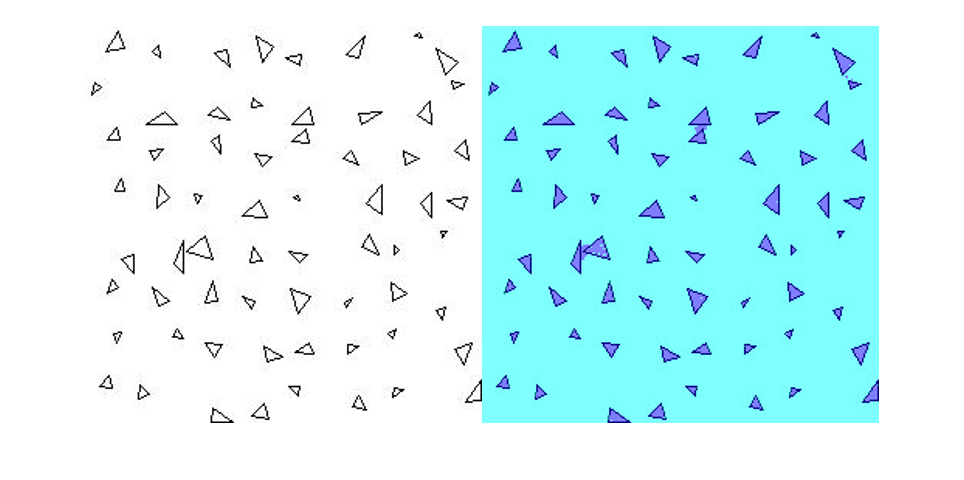Segment the test image using `semanticseg` and display the results using `labeloverlay`.

```C = semanticseg(imgTest,net); B = labeloverlay(imgTest,C); figure imshow(B)```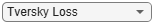## Input Arguments

collapse all

Input image, specified as one of the following.

Image TypeData Format
Single 2-D grayscale image2-D matrix of size H-by-W
Single 2-D color image or 2-D multispectral image3-D array of size H-by-W-by-C. The number of color channels C is 3 for color images.
Series of P 2-D images4-D array of size H-by-W-by-C-by-P. The number of color channels C is 1 for grayscale images and 3 for color images.
Single 3-D grayscale image with depth D3-D array of size H-by-W-by-D
Single 3-D color image or 3-D multispectral image4-D array of size H-by-W-by-D-by-C. The number of color channels C is 3 for color images.
Series of P 3-D images5-D array of size H-by-W-by-D-by-C-by-P

The input image can also be a `gpuArray` (Parallel Computing Toolbox) containing one of the preceding image types (requires Parallel Computing Toolbox™).

Data Types: `uint8` | `uint16` | `int16` | `double` | `single` | `logical`

Network, specified as a `SeriesNetwork` (Deep Learning Toolbox), `DAGNetwork` (Deep Learning Toolbox), or `dlnetwork` (Deep Learning Toolbox) object.

Region of interest, specified as one of the following.

Image TypeROI Format
2-D image4-element vector of the form [x,y,width,height]
3-D image6-element vector of the form [x,y,z,width,height,depth]

The vector defines a rectangular or cuboidal region of interest fully contained in the input image. Image pixels outside the region of interest are assigned the <`undefined`> categorical label. If the input image consists of a series of images, then `semanticseg` applies the same `roi` to all images in the series.

Collection of images, specified as a datastore. The `read` function of the datastore must return a numeric array, cell array, or table. For cell arrays or tables with multiple columns, the function processes only the first column.

### Name-Value Pair Arguments

Specify optional comma-separated pairs of `Name,Value` arguments. `Name` is the argument name and `Value` is the corresponding value. `Name` must appear inside quotes. You can specify several name and value pair arguments in any order as `Name1,Value1,...,NameN,ValueN`.

Example: `'ExecutionEnvironment'`,`'gpu'`

Returned segmentation type, specified as `'categorical'`, `'double'`, or `'uint8'`. When you specify `'double'` or `'uint8'`, the function returns the segmentation results as a label array containing label IDs. The IDs are integer values that correspond to the class names defined in the classification layer used in the input network.

You cannot use the `OutputType` property with an `ImageDatastore` object input.

Group of images, specified as an integer. Images are grouped and processed together as a batch. Batches are used for processing a large collection of images and they improve computational efficiency. Increasing the '`MiniBatchSize`' value increases the efficiency, but it also takes up more memory.

Hardware resource for processing images with a network, specified as `'auto'`, `'gpu'`, or `'cpu'`.

ExecutionEnvironmentDescription
`'auto'`Use a GPU if available. Otherwise, use the CPU. The use of GPU requires Parallel Computing Toolbox and a CUDA® enabled NVIDIA® GPU. For information about the supported compute capabilities, see GPU Support by Release (Parallel Computing Toolbox).
`'gpu'`Use the GPU. If a suitable GPU is not available, the function returns an error message.
`'cpu'`Use the CPU.

Performance optimization, specified as `'auto'`, `'mex'`, or `'none'`.

AccelerationDescription
`'auto'`Automatically apply a number of optimizations suitable for the input network and hardware resource.
`'mex'`Compile and execute a MEX function. This option is available when using a GPU only. You must also have a C/C++ compiler installed. For setup instructions, see MEX Setup (GPU Coder).
`'none'`Disable all acceleration.

The default option is `'auto'`. If you use the `'auto'` option, then MATLAB does not ever generate a MEX function.

Using the `'Acceleration'` options `'auto'` and `'mex'` can offer performance benefits, but at the expense of an increased initial run time. Subsequent calls with compatible parameters are faster. Use performance optimization when you plan to call the function multiple times using new input data.

The `'mex'` option generates and executes a MEX function based on the network and parameters used in the function call. You can have several MEX functions associated with a single network at one time. Clearing the network variable also clears any MEX functions associated with that network.

The `'mex'` option is only available when you are using a GPU. Using a GPU requires Parallel Computing Toolbox and a CUDA enabled NVIDIA GPU. For information about the supported compute capabilities, see GPU Support by Release (Parallel Computing Toolbox). If Parallel Computing Toolbox or a suitable GPU is not available, then the function returns an error.

`'mex'` acceleration does not support networks specified as a `dlnetwork` (Deep Learning Toolbox) object.

`'mex'` acceleration does not support all layers. For a list of supported layers, see Supported Layers (GPU Coder).

Classes into which pixels or voxels are classified, specified as `'auto'`, a cell array of character vectors, a string vector, or a categorical vector. If the value is a categorical vector Y, then the elements of the vector are sorted and ordered according to `categories``(Y)`.

If the network is a `dlnetwork` (Deep Learning Toolbox) object, then the number of classes specified by '`Classes`' must match the number of channels in the output of the network predictions. By default, when '`Classes`' has the value `'auto'`, the classes are numbered from 1 through C, where C is the number of channels in the output layer of the network.

If the network is a `SeriesNetwork` (Deep Learning Toolbox) or `DAGNetwork` (Deep Learning Toolbox) object, then the number of classes specified by '`Classes`' must match the number of classes in the classification output layer. By default, when '`Classes`' has the value `'auto'`, the classes are automatically set using the classification output layer.

Folder location, specified as `pwd` (your current working folder), a string scalar, or a character vector. The specified folder must exist and have write permissions.

This property applies only when using an `ImageDatastore` object input.

Prefix applied to output file names, specified as a string scalar or character vector. The image files are named as follows:

• `prefix_N.png`, where `N` corresponds to the index of the input image file, `imds.Files`(N).

This property applies only when using an `ImageDatastore` object input.

Display progress information, specified as `'true'` or `'false'`.

This property applies only when using an `ImageDatastore` object input.

## Output Arguments

collapse all

Categorical labels, returned as a categorical array. The categorical array relates a label to each pixel or voxel in the input image. The images returned by `readall`(`datastore`) have a one-to-one correspondence with the categorical matrices returned by `readall`(`pixelLabelDatastore`). The elements of the label array correspond to the pixel or voxel elements of the input image. If you select an ROI, then the labels are limited to the area within the ROI. Image pixels and voxels outside the region of interest are assigned the <`undefined`> categorical label.

Image TypeCategorical Label Format
Single 2-D image2-D matrix of size H-by-W. Element `C`(i,j) is the categorical label assigned to the pixel `I`(i,j).
Series of P 2-D images3-D array of size H-by-W-by-P. Element `C`(i,j,p) is the categorical label assigned to the pixel `I`(i,j,p).
Single 3-D image3-D array of size H-by-W-by-D. Element `C`(i,j,k) is the categorical label assigned to the voxel `I`(i,j,k).
Series of P 3-D images4-D array of size H-by-W-by-D-by-P. Element `C`(i,j,k,p) is the categorical label assigned to the voxel `I`(i,j,k,p).

Confidence scores for each categorical label in `C`, returned as an array of values between `0` and `1`. The scores represents the confidence in the predicted labels `C`. Higher score values indicate a higher confidence in the predicted label.

Image TypeScore Format
Single 2-D image2-D matrix of size H-by-W. Element `score`(i,j) is the classification score of the pixel `I`(i,j).
Series of P 2-D images3-D array of size H-by-W-by-P. Element `score`(i,j,p) is the classification score of the pixel `I`(i,j,p).
Single 3-D image3-D array of size H-by-W-by-D. Element `score`(i,j,k) is the classification score of the voxel `I`(i,j,k).
Series of P 3-D images4-D array of size H-by-W-by-D-by-P. Element `score`(i,j,k,p) is the classification score of the voxel `I`(i,j,k,p).

Scores for all label categories that the input network can classify, returned as a numeric array. The format of the array is described in the following table. L represents the total number of label categories.

Image TypeAll Scores Format
Single 2-D image3-D array of size H-by-W-by-L. Element `allScores`(i,j,q) is the score of the qth label at the pixel `I`(i,j).
Series of P 2-D images4-D array of size H-by-W-by-L-by-P. Element `allScores`(i,j,q,p) is the score of the qth label at the pixel `I`(i,j,p).
Single 3-D image4-D array of size H-by-W-by-D-by-L. Element `allScores`(i,j,k,q) is the score of the qth label at the voxel `I`(i,j,k).
Series of P 3-D images5-D array of size H-by-W-by-D-by-L-by-P. Element `allScores`(i,j,k,q,p) is the score of the qth label at the voxel `I`(i,j,k,p).

Semantic segmentation results, returned as a `pixelLabelDatastore` object. The object contains the semantic segmentation results for all the images contained in the `ds` input object. The result for each image is saved as separate `uint8` label matrices of PNG images. You can use `read`(`pxds`) to return the categorical labels assigned to the images in `ds`.

The images in the output of `readall`(`ds`) have a one-to-one correspondence with the categorical matrices in the output of `readall`(`pxds`).

## Extended Capabilities

### External Websites

Introduced in R2017b